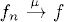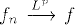# CONVERGENCE

In measure theory, there exist several different notions of convergence of measurable functions, for example, convergence almost everywhere, convergence in measure, convergence in Lp, to just name a few. In this chapter, we present definitions of some common notions of convergence.

# 9.1 Basic Concepts and Facts

Definition 9.1 (Convergence in Measure). Let f, f1, f2, ··· be Borel measurable functions on a measure space (S, ∑, μ). The sequence {fn}n≥1 is said to converge to f in measure, written as, if and only if for every> 0, μ{s : |fn(s) − f(s)| ≥} → 0 as n → ∞.

In particular, if μ is a probability measure, then convergence in measure is also referred to as convergence in probability.

Definition 9.2 (Convergence in Lp). Let f be a Borel measurable function on a measure space (S, ∑, μ) and {fn}n≥1 a sequence of of functions in Lp(S, ∑, μ). The sequence {fn}n≥1 is said to converge to f in Lp, written as, if and only if ||fnf||p → 0 as n → ∞, where.

Definition ...

Get Measure, Probability, and Mathematical Finance: A Problem-Oriented Approach now with the O’Reilly learning platform.

O’Reilly members experience live online training, plus books, videos, and digital content from nearly 200 publishers.